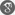##### Cite as:
Devendra Kumar, Slow Growth and Optimal Approximation of Pseudoanalytic Functions on the Disk, Int. J. Anal. Appl., 2 (1) (2013), 26-37.

#### Abstract

Pseudoanalytic functions (PAF) are constructed as complex combination of real-valued analytic solutions to the Stokes-Betrami System. These solutions include the generalized biaxisymmetric potentials. McCoy  considered the approximation of pseudoanalytic functions on the disk. Kumar et al.  studied the generalized order and generalized type of PAF in terms of the Fourier coefficients occurring in its local expansion and optimal approximation errors in Bernstein sense on the disk. The aim of this paper is to improve the results of McCoy  and Kumar et al. . Our results apply satisfactorily for slow growth.

#### References

1. K.W. Bauer and St. Ruschewyh, Differential Operators for Partial Differential Equations and Function Theoretic Methods, Lecture Notes in Math. No. 791, Springer, New York, 1980.2. H. Bavinck, Jacobi Series and Approximation, Mathematical Center Tracts No. 39, Mathematisch Centrum, Amsterdam, 1972.3. S. Bergman, Integral operators in the Theorey of Linear Partial Differential Equations, (Berlin Springer-Verlag), 1969.4. L. Bers, Theory of Pseudoanalytic Functions, Lecture Notes (New York: New York University), 1953.5. R.P. Gilbert, Function Theoretic Methods in Partial Differential Equations, (New York: Academic Press), 1969.6. G.P. Kapoor and A. Nautiyal, Growth and approximation of generalized bi-axillay symmetric potentials, Indian J. Pure Appl. Math. 19(5)(1988), 464-476.7. G.P. Kapoor and A. Nautiyal, Polynomial approximation of an entire function of slow growth, J. Approx. Theory 32, (1981), 64-75.8. D. Kumar, Ultraspherical expansions of generalized bi-axially symmetric potentials and pseudoanalytic functions, Complex Variables and Elliptic Equations, 53(1) (2008), 53-64.9. D. Kumar, Vandna Jain and Balbir Singh, Generalized growth and approximation of pseudo analytic functions on the disk,British Journal of Mathematics and Computer Science,in press.10. P.A. McCoy, Approximation of pseudoanalytic functions on the unit disk, Complex Variables and Elliptic Equations, 6(2)(1986), 123-133.11. I. Vekua, Generalized Analytic Functions (Reading, M.A. Addison - Wesley), 1962.12. A. Weinstein, Transonic flow and generalized axially symmetric potential theory, Proc. NOL Aeroballistic Res. Symp. Naval Ord Laboratory, White Oak, MD, 1949.